# Boost Converter Not Boosting

#### IBO3252

Joined May 17, 2023
4
Hi Guys,

I'm designing a boost converter and using irf44zn N-mosfet as a switch and Tlp350 to drive the mosfet the following parameters )50% duty cycle, 50khz Fq, and Vin 18 volt Vout 24 volt, 470 microfarad, 1 K ohm load and inductance of 132 microH one in a bread boorad (Circuit shemtici is in pic-4)

The problem is that the circuit doesn't work until I touch the Drain & the Gate of the Mosfet together which I think shorts the circuit and then I get the targeted Vout but i get this wearied reading from the Voltage source see (pic -1).Moreover, Although the Oscilloscope show the pk-pk Vout is about 24 but when I touch the voltmeter to the load legs, I get around 0.4 Volts which very strange hence it reading a different value do anyone can help plz

Note: I'm using Tiva C tm4c123gh6pm launch pad to generate the pwm signals

pic-1 :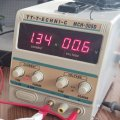pic-2 :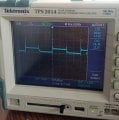pic-3 :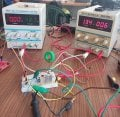pic-4 :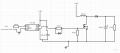#### Ian0

Joined Aug 7, 2020
8,947
Not the easiest thing to spot on the datasheet, but the TLP350 has an undervoltage lockout which can be as high as 13.5V.
Does it work if you increase the supply to 14V?

#### IBO3252

Joined May 17, 2023
4
Not the easiest thing to spot on the datasheet, but the TLP350 has an undervoltage lockout which can be as high as 13.5V.

Does it work if you increase the supply to 14V?
Hi Ian0, Thanks for your response,

I just figured out the problem wasn't about the voltage, although it's Tlp related, it was in the Tlp350 poor connection wires to other components so I couldn't drive the gate correctly So again thanks Ian.

But Now I'm trying to adjust the load current to a specific value
My new parameters are
Vin = 7 Volts
Vout = 15 Volts
R = 81ohm ceramic resistor that can withstand 11W (this value is obtained from 33 + 33 +15 resistors in series)
C = 48 μ F
L = 620 μ H
Duty cycle =58%
using the IN5819 that has voltage drop= 0,6 volt
Now according to my calculation, I should get 0,3 Amps load current but I'm getting 0.43 Amps & Vout of 14 to 14.2 volts

How can I get the calculated 0.3 Amps?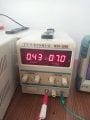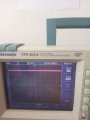#### arhurjs

Joined Feb 27, 2020
3
The operation of your circuit is satisfactory. However, you will never achieve the ideal input current because there are losses in the MOSFET, diode, inductor, and capacitors.

You have calculated the input power as follows: Pin = 7V * 0.43A = 3.01W, and the output power as Pout = 14.2V * 0.175A = 2.48W.

The efficiency (η) of your circuit can be calculated as the ratio of output power to input power: η = Pout / Pin = 2.48W / 3.01W = 0.82, which is equal to 82%.

This means that 82% of the input power is being converted into output power, while the remaining 18% is lost due to the inherent losses in the MOSFET, diode, inductor, and capacitors.

•IBO3252